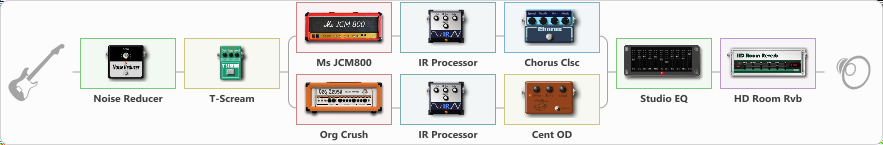# Bark At The Moon/Ozzy

Discussion in 'ToneLib-GFX presets' started by Tucker Stanley, Feb 5, 2023.

1. Bark At The Moon/Ozzy

Preset name: Jake E Lee

Effects chain:Effect: "Noise Reducer" (Dynamics / Filter), active - "yes"
{
"Sens" = 65
"Mode" = Hard
}

Effect: "T-Scream" (Overdrive / Distortion), active - "yes"
{
"Drive" = 74
"Tone" = 41
"Level" = 50
}

Effect: "Splitter" (Dynamics / Filter)
{
"A-Bypass" = Off
"A-Pan" = 0
"A-Level" = 55
"B-Bypass" = Off
"B-Pan" = 0
"B-Level" = 55
"Width" = 0

'A' branch:
{

Effect: "Ms JCM800" (Amp simulators), active - "yes"
{
"Gain" = 70
"Bass" = 68
"Middle" = 45
"Treble" = 36
"Presence" = 29
"Master" = 55
"Level (dB)" = -3
}

Effect: "IR Processor" (Cabinets), active - "yes"
{
"IR" = Cab IR Sample4
"Low Cut (Hz)" = 125
"Hi Cut (kHz)" = 6.5
"Mix" = 100
"Level (dB)" = 3
}

Effect: "Chorus Clsc" (Modulation / Sfx), active - "yes"
{
"Speed" = 0.7
"Depth" = 25
"Center" = 3.9
"Mode" = Stereo
}
}
'B' branch:
{

Effect: "Org Crush" (Amp simulators), active - "yes"
{
"Gain" = 56
"Bass" = 67
"Middle" = 55
"Treble" = 19
"Presence" = 35
"Master" = 55
"Level (dB)" = -2
}

Effect: "IR Processor" (Cabinets), active - "yes"
{
"IR" = Cab IR Sample4
"Low Cut (Hz)" = 175
"Hi Cut (kHz)" = 6.5
"Mix" = 100
"Level (dB)" = 0
}

Effect: "Cent OD" (Overdrive / Distortion), active - "yes"
{
"Drive" = 40
"Tone" = 76
"Level" = 38
}
}
}

Effect: "Studio EQ" (Dynamics / Filter), active - "yes"
{
"31 Hz" = -12
"62 Hz" = -14
"125 Hz" = 2
"250 Hz" = -5
"500 Hz" = -6
"1 kHz" = -4
"2 kHz" = 5
"4 kHz" = 5
"8 kHz" = -3
"16 kHz" = -11
"Level (dB)" = -1
}

Effect: "HD Room Rvb" (Reverberation), active - "yes"
{
"Time" = 2.5
"PreLPF" = 0
"PreDelay" = 0
"HiDamp" = 100
"LoGain" = -12.0
"Mix" = 25
}

Note: You will need to download and install the ToneLib-GFX software to use the preset.

#### Attached Files:

• ###### Ozzy.tlgfx
File size:
7.5 KB
Views:
3,230
BK216 likes this.